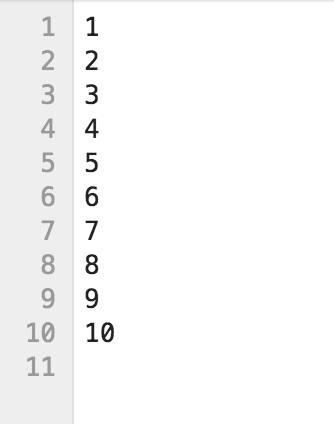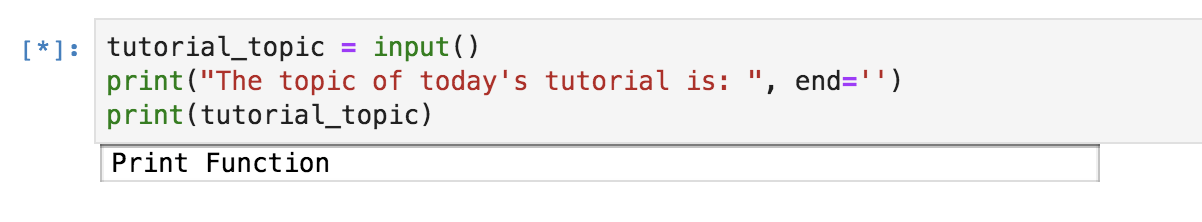Tutorials
must read
python

# Python Print() Function

Learn how you can leverage the capability of a simple Python Print function in various ways with the help of examples.

If you are just getting started in Python and would like to learn more, take DataCamp's Introduction to Data Science in Python course.A lot of you, while reading this tutorial, might think that there is nothing undiscovered about a simple Python Print function since you all would have started learning Python with the evergreen example of printing Hello, World!. It's also true that Python or, for that matter, any programming language, the Print function is the most basic and the baby step that you take while learning a particular language. However, while learning a programming language, you sometimes tend to focus on more advanced topics and many times forget the intricacies or capabilities of a simple function that you might almost always use.

The focus of today's tutorial will be entirely on Python's Print function; you will learn about the most underestimated function.

Let's begin this tutorial by printing the Hello, World! example.

print("Hello, World!")

Hello, World!


Unlike Python2, which did not require you to put a parenthesis, in Python3, parenthesis is a must else it will raise a syntax error as shown below.

print "Hello, World!"

  File "<ipython-input-6-a1fcabcd869c>", line 1
print "Hello, World!"
^
SyntaxError: Missing parentheses in call to 'print'. Did you mean print("Hello, World!")?


From the above output, you can conclude that in Python3, print() is not a statement but a function.

Having said that, let's check the type/class of the print() function.

type(print)

builtin_function_or_method


It returns builtin_function_or_method, which means it is a predefined or a builtin Python Function.

Now let's say you want to add a new line or a vertical space between the below two outputs, to achieve this you can simply call the print() function without passing any argument in it.

print("Hello, World!");print("Hello, World!")

Hello, World!
Hello, World!

print("Hello, World!")
print()
print("Hello, World!")

Hello, World!

Hello, World!


Let's look at the syntax of the print() function. print(value, ..., sep=' ', end='\n', file=sys.stdout, flush=False) As you know by now, the print function Prints the values to a stream, or to sys.stdout by default. The sys.stdout or system standard output means the print function will print the output on the screen, and it can even be changed to stdin or stderr.

#### Optional keyword arguments:

• sep: It can be a string which you would like to insert between values, defaults to space.

Let's put a list of words inside the print function and separate them with a new line. Remember, by default; the separator adds a blank space between each word.

print('datacamp','tutorial','on','python','print','function')

datacamp tutorial on python print function

print('datacamp','tutorial','on','python','print','function',sep='\n') #\n will put each word in a new line

datacamp
tutorial
on
python
print
function


Similarly, you can separate them with a comma, or add two \n which will add an empty line in between or even add a plus $(+)$ sign.

print('datacamp','tutorial','on','python','print','function',sep=',')

datacamp,tutorial,on,python,print,function

print('datacamp','tutorial','on','python','print','function',sep='\n\n')

datacamp

tutorial

on

python

print

function

print('datacamp','tutorial','on','python','print','function',sep=',+')

datacamp,+tutorial,+on,+python,+print,+function


Before you jump on to the next argument, i.e., end, remember that you can also pass in a variable to the print function. To understand this, let's take an example by defining a list of integers and pass it to the print function. You would expect it to print the list of integers.

int_list = [1,2,3,4,5,6]
print(int_list)

[1, 2, 3, 4, 5, 6]

• end: It is a string appended after the last value, defaults to a newline. It allows the programmer to define a custom ending character for each print call other than the default newline or \n.

Let's say you have two strings and you want to join them together with space, all you would need to do is in the print function of the first string str1 add end argument with quotes, and you can expect the two strings to be joined by a space.

str1 = 'datacamp tutorial on'
str2 = 'python print function'

print(str1)
print(str2)

datacamp tutorial on
python print function

print(str1,end=' ')
print(str2)

datacamp tutorial on python print function


Let's take another example where you have a function whose job is to print all values within a list on the same line. You could achieve this with the end argument, as shown below:

def value(items):
for item in items:
print(item, end=' ')

value([1,2,3,4])

1 2 3 4

• file: A file-like object (stream); defaults to the current sys.stdout. Here you can mention the file in which you would like to write or append the output of the print function.

By using the file argument, you can store the output of the print function to a file in various formats like .csv, .txt, etc. Let's understand this by taking an example wherein you iterate over each element of the list. It is saved in a text file. To accomplish this, first, you will open a text file in an append state in which you will be saving the output of the print statement. Next, you will define the function whose output will be appended in the text file.

file = open('print.txt','a+')

def value(items):
for item in items:
print(item, file=file)
file.close() # close the file ones the function execution completes.

value([1,2,3,4,5,6,7,8,9,10])


Once the above function execution completes, you should see a file named print.txt in your current directory. Let's open it and see its contents.From the above output, it is clear that you can even save the output to files and not just print the output on the terminal or within the notebook.

• flush: It determines whether to forcibly flush the stream. By default, it is set to False.

Typically, output to a file or the console is buffered, with text output at least until you print a newline (Source). Buffer means that the output is stored in some kind of a register where the output resides before it is ready to be stored or the file is finally closed. The job of the flush is to make sure that the output, which is being buffered, goes to the destination safely.

import time
print('Please enter your email-id : ', end=' ')
#print('Please enter your email-id : ', end=' ', flush=True) #run this to see the difference and comment out the above line of code.
time.sleep(5)

Please enter your email-id :


If you run the above lines of code in the terminal, you will notice that the prompt string does not show up until the sleep timer ends and the program exits (Source). However, if you add flush=True in the print function, you will see the prompt, and then you will have to wait for 5 seconds for the program to finish.

Somehow the Jupyter Notebook or Jupyter Lab takes care of it and shows the prompt before the 5 seconds timer, so if you want to test this functionality of print, make sure to run it in command line terminal and not on the jupyter notebook.

Let's now see how you can make use of the print function to take input from the user in the jupyter notebook. For this, you will use python's builtin function input().

tutorial_topic = input()
print("The topic of today's tutorial is: ", end='')
print(tutorial_topic)

 Print Function

The topic of today's tutorial is: Print Function


As soon as you run the above cell, you should see an output as shown below:Here you defined an optional named argument end that you learned before, which concatenates the static statement inside print statement with the user input.

Let's see some cooler ways of printing variable values inside the print function.

• To display a variable's value along with a predefined string, all you need to do is add a comma in between the two. Here the position of the predefined string and the variable does not matter.
a = 2
b = "Datacamp"
print(a,"is an integer while",b,"is a string.")

2 is an integer while Datacamp is a string.

• You can make use of the format argument wherein you can pass as many variables as you want to print. When you pass the variables in the format function, you need to specify the index numbers (order in which they are placed inside the format argument) in the predefined string. So this print function will now act as a template for you.

Another thing to remember is that here the index numbers are represented with a curly bracket {} representing a placeholder.

Let's understand it with the below example:

a = 2
b = "Datacamp"
print("{0} is an integer while {1} is a string.".format(a,b))

2 is an integer while Datacamp is a string.


If you put the index number of the second variable at both places, as expected, it will print the same values, in this case, b variable for both.

print("{1} is an integer while {1} is a string.".format(a,b))

Datacamp is an integer while Datacamp is a string.

• Similar to a format argument where your print function acts as a template, you have a percentage (%) sign that you can use to print the values of the variables.

Like format argument, this also has a concept of placeholders. However, unlike the format function where you pass in just the index numbers, in this, you also need to specify the datatype the placeholder should expect.

• %d is used as a placeholder for numeric or decimal values.
• %s is used as a placeholder for strings.
a = 2
b = "Datacamp"
print("%d is an integer while %s is a string."%(a,b))

2 is an integer while Datacamp is a string.


Let's see what happens if you specify %s for the variable a which is an integer.

print("%s is an integer while %s is a string."%(a,b))

2 is an integer while Datacamp is a string.


As you can see from the above example, it still works. The reason is that the Print function implicitly performed typecasting and converted the integer to a string. However, the opposite is not true. It will fail to convert a string to an integer and will result in a TypeError.

Let's find out.

print("%d is an integer while %d is a string."%(a,b))

---------------------------------------------------------------------------

TypeError                                 Traceback (most recent call last)

<ipython-input-121-68c55041ecfe> in <module>
----> 1 print("%d is an integer while %d is a string."%(a,b))

TypeError: %d format: a number is required, not str


## Conclusion

Congratulations on finishing the tutorial.

This tutorial was a good starting point for beginners who aspire to become proficient in Python. You might want to play around with the Print function a bit more and explore a few more functionalities of it that might have been missed out in this tutorial.

Please feel free to ask any questions related to this tutorial in the comments section below.

If you are just getting started in Python and would like to learn more, take DataCamp's Introduction to Data Science in Python course.